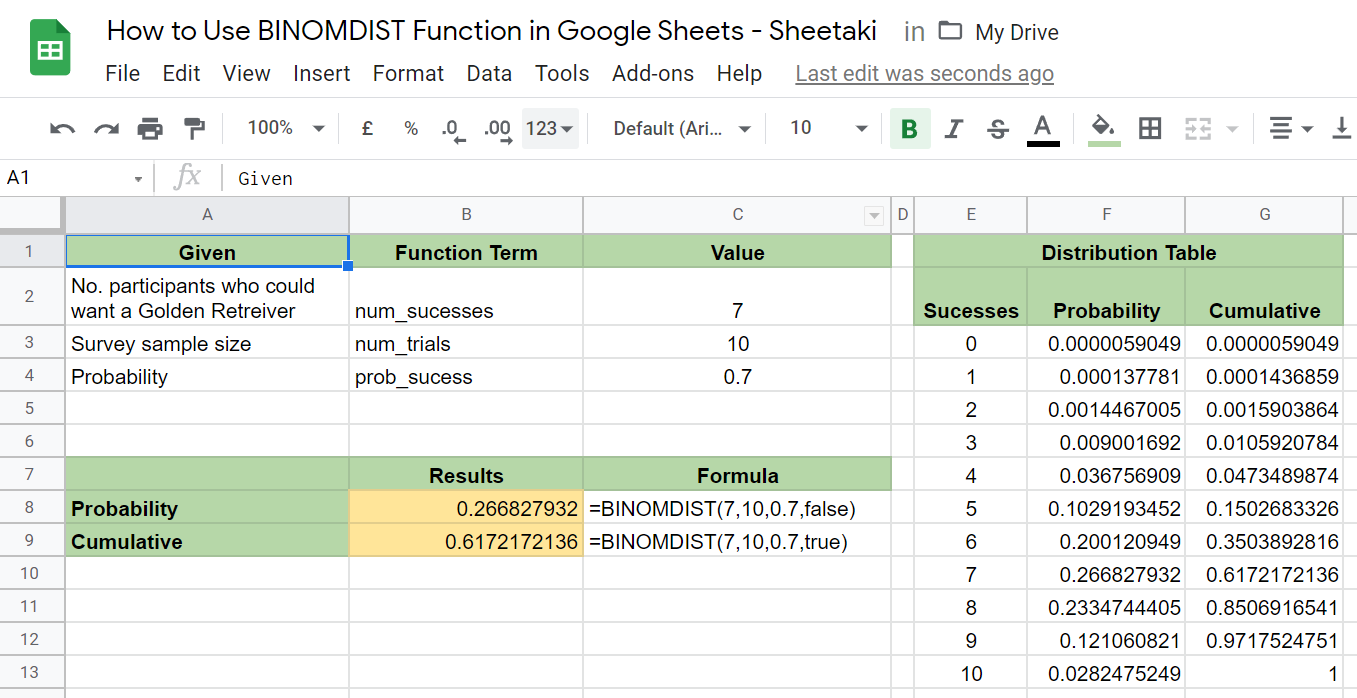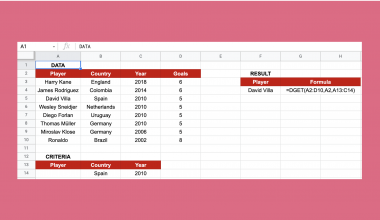How to Use BINOMDIST Function in Google Sheets

The BINOMDIST function in Google Sheets calculates for the binomial distribution or cumulative distribution of the probability.

The binomial takes into account binary events or situations with only two possible outcomes. A binomial distribution is the likelihood of success or failure of an outcome repeated or observed multiple times in trials. A cumulative distribution, on the other hand, is the maximum number of successful trials.

The rules for calculating binomial distribution in Google Sheets are as follows:

• There should be a fixed number of trials provided.
• There should be only two possible outcomes for all trials. Meaning, the result could be either positive or negative.
• Each trial should be independent, and so their outcomes should not affect each other.

Let’s take an example.

A simple situation with the criteria stated above would determine how likely you could get 5 heads and 3 tails in your 8 tosses. Here, the fixed number of trials is 8. Then, the probability of getting head on each trial is 50% or 0.50. And the number of successes (getting heads) to check the probability of is 5.

Given that, you are 21.88% likely to get 5 heads out of 8 coin tosses. We’ll get into detail about how we got this answer later on.

With Google Sheets and an understanding of the BINOMDIST Function, you can answer this question and many others following the conditions outlined in bullet points earlier. You can also imagine that this would be helpful with problem solving during your math class.

So, buckle up, and get to know how to use the BINOMDIST function in Google Sheets through this article. We’ll explain our process step-by-step and we even have a sample spreadsheet that you can make a copy of to guide you even better.

The Anatomy of the BINOMDIST Function in Google Sheets

First, let’s get to know the syntax (the way we write) of the BINOMDIST function. This goes as follows:

=BINOMDIST(num_successes, num_trials, prob_success, cumulative)

Let’s dissect this thing and understand what each of these terms means:

• = the equal sign is just how we start any function in Google Sheets
• BINOMDIST () is our binomial probability distribution function.
• num_successes is the number of successful trials.
• num_trials is the number of trials total.
• prob_sucess is the probability of success.
• cumulative is either “true” or “false”. If you input “true”, you’re asking the formula to do a cumulative sum of the probabilities. This is “false” by default, which generates the probability mass function.

Note that you will get the #VALUE! error value if your num_successes, num_trials, prob_success, are non-numeric. On the other hand, you will get the #NUM! error value in two cases. One, if your prob_success is greater than 0 or more than. Two, if your num_successes is less than 0 or breather than your num_trials.

A Real Example of Using the BINOMDIST Function in Google Sheets

Now, let’s take a look at the example below to see how to use the BINOMDIST function in Google Sheets.In this situation, I’m doing a survey on what breed of dogs that homeowners with children would prefer to have in their household. I have a sample size of 60, and I know that 70% of the entire population would opt to have a Golden Retriever. With that, I’m trying to figure out the probability that 35 out of my 60 survey participants would say they want a Golden Retriever.

This is the same way we get the 21.88% probability to get 5 heads out of 8 coin tosses mentioned in the example up in the introduction of this article.

The results give you decimals. To get the percentage, you simply move two decimal places to the left and as standard, including the decimal places to the right up to the hundreds.

Now, let’s clarify the difference between the probability and cumulative results. When the cumulative part of the function is set to default or ‘false’, we get the probability of a definite number of binary outcomes. When it is set to ‘true’, the formula calculates the maximum probability of a number of successes in a given number of trials.

In the chart, you’ll see, the probabilities are summed to result in the cumulative probability up to that point. This is useful when the problem asks for probability of at least or at most a certain number instead of exact.

At this point, we will now get into the steps of using the BINOMDIST function given the example situation I have presented.

How to Use the BINOMDIST Function In Google Sheets

1. First, select any cell to make it active. For this guide, I’ve written down the given to my problem on the Google Sheet and I’ve designated F3 to be where my first result goes. You don’t have to encode everything down the way I did if you only want the results.2. Next, simply type the equal sign ‘=‘ to begin the function and then follow it with the name of the function which is ‘BINOMDIST‘ (or ‘BINOMDIST, whichever works). While you’re typing, an auto-suggest box will appear with the BINOMDIST and the BINOM.DIST functions, among other binomial formulas. They both work the same, actually, but here, I chose BINOMDIST.3. Then, input all the needed items accordingly and separate them with commas. For now, use ‘false’ as the cumulative. This will show the probability of a certain outcome happening given the inputted number of trials.4. Now, chose another cell and repeat steps 1 to 5 but this time, choose ‘true’ as the cumulative. This will show the sum of the probabilities up to the inputted number of successes.You’ve just learned how to use the BINOMDIST function in Google Sheets. Get to know other Google Sheets formulas so that you can make the most out of this wonderful tool. Also, make sure to subscribe to be notified of our future posts.Our goal this year is to create lots of rich, bite-sized tutorials for Google Sheets users like you. If you liked this one, you'll love what we are working on! Readers receive ✨ early access ✨ to new content.

D

You May Also LikeHow to Use COMBIN Function in Google Sheets

The COMBIN function in Google Sheets helps you quickly calculate the estimated number of groups for a specific…How to Count the Number of Words in Google Sheets

Knowing how to count the number of words in Google Sheets is useful if you need to know…How to Find the Highest N Values in Each Group in Google Sheets

This guide to find the highest N Values in each group in Google Sheets is useful if you…How to Use DGET Function in Google Sheets

The DGET function in Google Sheets is useful if you need a single value from a database table-like…